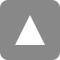# Department of Statistical Inference and Mathematics

The Department of Statistical Inference and Mathematics carries out research into general statistical theory, statistical learning theory, optimization, and algorithms for statistical inference.

## Mathematical Statistics Group

The Mathematical Statistics Group is concerned with aspects of statistical inference theory, modeling of uncertain phenomena, stochastic processes and their application to inference, probability and distribution theory, and the related mathematics.

## Learning and Inference Group

The Learning and Inference Group develops statistical methodologies to describe the stochastic structure of data mathematically and clarify the potential and the limitations of the data theoretically.

## Mathematical Optimization Group

The Mathematical Optimization Group focuses on mathematical theory and practical applications of optimization and computational algorithms together with underlying numerical or functional analysis and discrete mathematics.